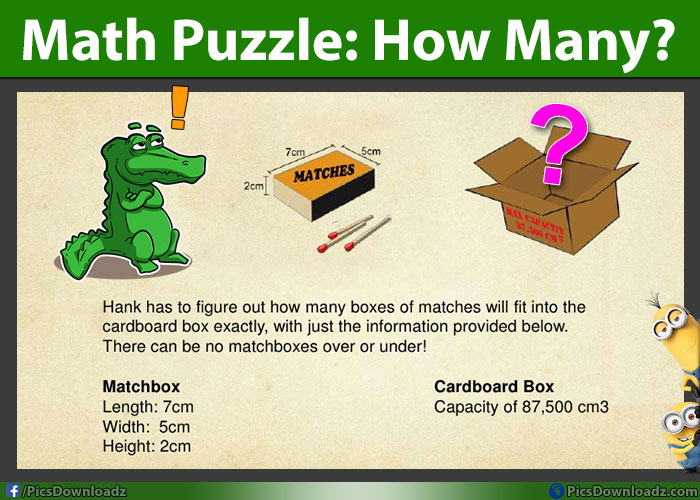This post may contain affiliate links. This means if you click on the link and purchase the item, We will receive an affiliate commission at no extra cost to you. See Our Affiliate Policy for more info.

How many boxes of matches will fit? – Fun Math Puzzles With Answer

0
691

Math Puzzles Only for Geniuses with Answer. Solve this Math Puzzle Problem!

It good to get some math knowledge with fun. So, here we come with an interesting and simple math puzzle problem. Solve this puzzle and comment your answer below.

In this puzzle, you will learn about volume. Read the question carefully and try to solve or find the formula to solve this math problem. Check the question below;

Math Problem: You have to find out how many boxes of matches will fit into the cardboard box exactly, with just the information provided below. There can be no matchboxes over or under!

Match Box: Length= 7cm, width= 5cm, height= 2cm.

Cardboard box: capacity of 87,500 cm^3

Math Puzzle Problem Image:First, you will have to find out the volume of the matchbox; (length × width × height).

So, we get 7cm × 5cm × 2cm = 70cm³

The Cardboard Box: Capacity 87,500cm³

So, total number of matches will fit = 87,500cm³ / 70cm³ = 1250 Matchboxes

Hope you find this puzzle interesting. If you have some more puzzles and tricks, please share with us via comments. We will make a post on your puzzles with an image. You can share our puzzle pics with your friends and group.

LIKE us on facebook and get more, funny and interesting stories in your timeline;

Take Care, Enjoy!

Search items:

Math Puzzles, Interesting Math Puzzles, Math Problems, Find the Answer, Solve Math Puzzles, Genius Math Puzzles, Puzzles for Genius, Only for Genius, Brainteasers, Brain Teasers Puzzles, Math Riddles, Riddles, Fun Math Puzzles, Difficult Math Puzzles, Volume Math Puzzles, Only for Genius Puzzles, Puzzles Images, Puzzles for Facebook, Puzzles for Whatsapp, Share Puzzles Image, Math and Brainteasers Puzzles, Math and Riddles, Solve if you are Genius, Math Puzzles with Answer, Only for Geniuses, Brain Traning Puzzles, Confusing Puzzles, Riddles Website, Puzzles Blog, Math Games,